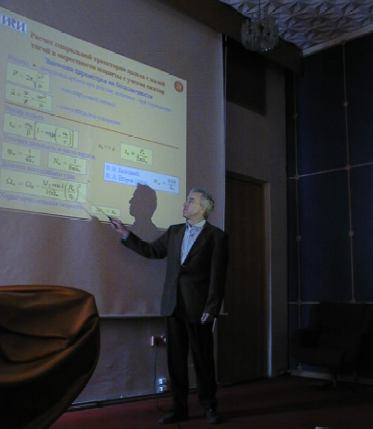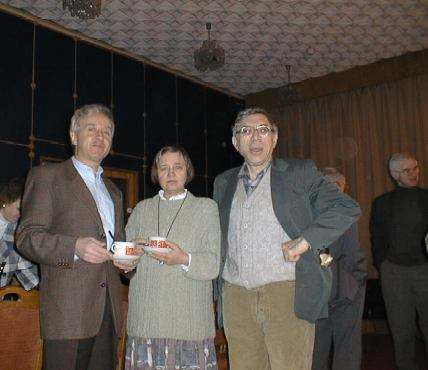A. A. Sukhanov
Modern Methods for Space Flight Dynamics and Their Applications

 Main page Reports Discussion The following effective mathematical methods are suggested for solution of different space flight dynamics problems: 1) A simple method for the calculation of the state transition matrices of the first and second order. The method allows calculation of the matrices for any orbit types. 2) A universal solution of the Lambert problem. The solution is given for all orbit types and for any number of complete revolutions. 3) A double planet swingby optimization. An optimization method for different cases of two sequential swingbys of a planet are considered. 4) Calculation of orbital parameters of a spiral low-thrust transfer near an oblate planet. Simple zeroth and first order formulas for the calculation of circle-to-circle, circle-to-infinity, and infinity-to-circle transfers are suggested. 5) Analytical optimization of an interplanetary low-thrust transfer. Approximate analytical expressions are given for calculation of the optimal low thrust vector and the spacecraft state vector for both solar and nuclear electric power. The transfer can include any number of planet swingbys and small body flybys. The suggested methods allow transfer trajectory design for both impulsive thrust and continuous one, and also solution of other astrodynamic problems. Applications of the methods are given. Photos